Latest Banking jobs   »   Quantitative Aptitude Quiz For IBPS Clerk...

# Quantitative Aptitude Quiz For IBPS Clerk Prelims 2021- 22nd October

Directions (1-5): Given below in each question there are two statements (I) and (II). You have to determine which statement is/are sufficient to give the answer of question. Also, there are five alternatives given, you have to choose one alternative as your answer of the questions.
(a) Statement I alone is sufficient to answer the question but statement II alone is not sufficient to answer the question.
(b) Statement II alone is sufficient to answer the question but statement I alone is not sufficient to answer the question.
(c) Both the statements taken together are necessary to answer the question, but neither of the statements alone is sufficient to answer the question.
(d) Either statement I alone or statement II alone is sufficient to answer the question.
(e) Statements I and II together are not sufficient to answer the question.

Q1. What will be the cost price of article?
I. Article is marked 50% above the cost price and discount allowed is Rs.400 more than the loss incurred on the article.
II. Ratio of discount allowed on article to selling price of the article is 21 : 39 and loss incurred on article is Rs.20.

Q2. Find the volume of the cone.
I. Slant height of the cone is 35cm and curved surface area of cone is 2310cm2.
II. Height of the cone is 7cm more than radius of the cone.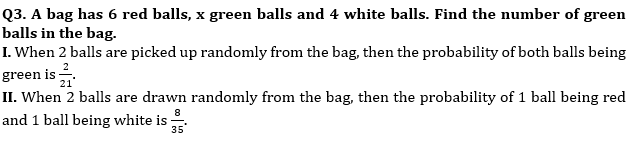Q4. A, B & C working together can complete a piece of work in 9 days. No. of days taken by A alone to complete the whole work.
I. B & C working together can complete same piece of work in 12 days.
II. B can complete same piece of work in 20 days and C is 20% more efficient than A.

Q5. Find speed of boat in still water.
I. A boat takes 8 hours to cover 40km in upstream and 60km in downstream.
II. Ratio of speed of boat in still water to speed of stream is 7 : 3.

Directions (6-10): The following questions are accompanied by two statements (I) and (II). You have to determine which statements(s) is/are sufficient/necessary to answer the questions.
(a) Statement (I) alone is sufficient to answer the question but statement (II) alone is not sufficient to answer the question.
(b) Statement (II) alone is sufficient to answer the question but statement (I) alone is not sufficient to answer the question.
(c) Both the statements taken together are necessary to answer the question, but neither of the statements alone is sufficient to answer the question.
(d) Either statement (I) or statement (II) by itself is sufficient to answer the question.
(e) Statements (I) and (II) taken together are not sufficient to answer the question.

Q6. What will be the speed of boat in still water?
I. Ratio of speed of boat in downstream to that of in upstream is 16 : 9.
II. Boat covers 80 km in downstream in 2.5 hours.

Q7. What will be the area of square?
I. Side of square is equal to breadth of rectangle, whose area and perimeter are 416 cm2 and 84 cm respectively and length is greater than breadth.
II. Ratio of length to breadth of rectangle is 13 : 8.

Q8. Find value of R.
I. Sameer invested Rs.5000 each at R% p.a. SI and R% p.a. CI respectively for 2 years. Total compound interest received is Rs. 112.5 more than total simple interest received by him.
II. Anurag invested Rs.X at R% p.a. at SI for 2 years and Rs.4000 at (R+5)% p.a. at SI for 3 years. Total interest received by him is Rs.4200.

Q9. Find profit percentage earned by shopkeeper on selling the article.
I. Ratio of cost price of an article to mark price of an article is 4 : 7 and ratio of discount allowed on article to profit earned on article is 49 : 11.
II. Discount allowed on article is Rs.190 more than profit earned on the article.

Q10. Find profit share of C at the end of the year.
I. A, B & C invested in a partnership business in the ratio 12 : 11 : 18 for 1 year. Difference between profit share of A & C at the end of the year is Rs.7800.
II. C, D & F invested in a partnership business in the ratio 5 : 8 : 2 and period of investment of C, D & F is 6 months, 4 months & 12 months. Profit share of F at the end of the year is Rs.18720.

Directions (11-15): In the given questions, two quantities are given, one as ‘Quantity I’ and another as ‘Quantity II’. You must determine relationship between two quantities and choose the appropriate option: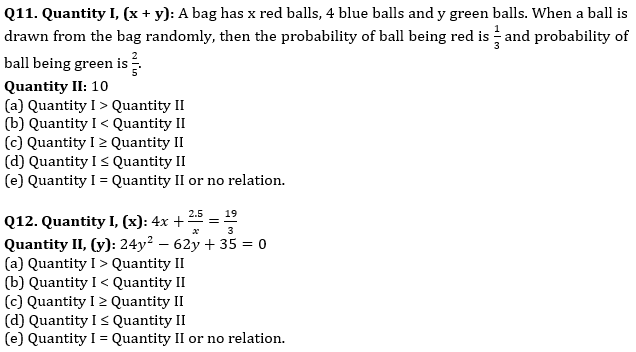Q13. Quantity I: A alone and B alone can complete a piece of work in 30 days and 40 days respectively. C is 20% less efficient than B. In how many days the same work will be completed, if A & C starts working together?
Quantity II: 20 days.
(a) Quantity I > Quantity II
(b) Quantity I < Quantity II
(c) Quantity I ≥ Quantity II
(d) Quantity I ≤ Quantity II
(e) Quantity I = Quantity II or no relation.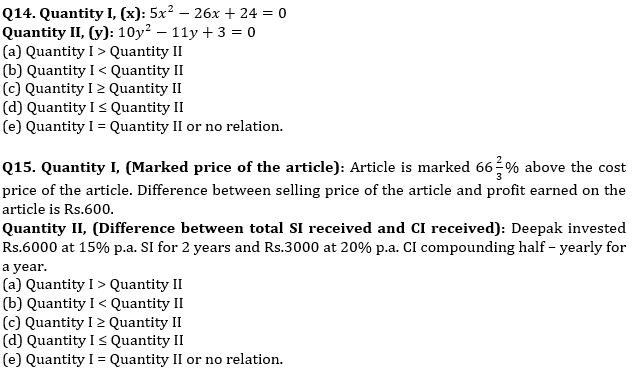Solutions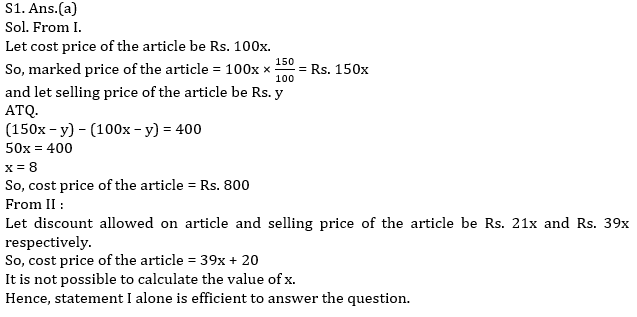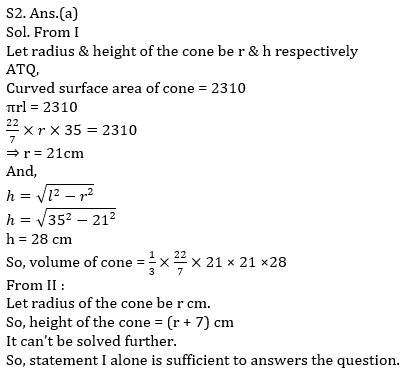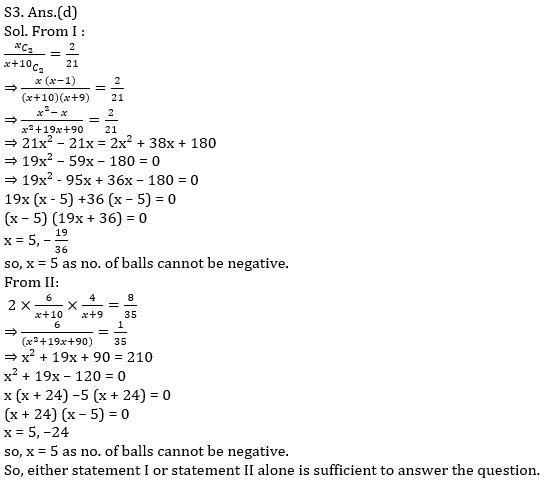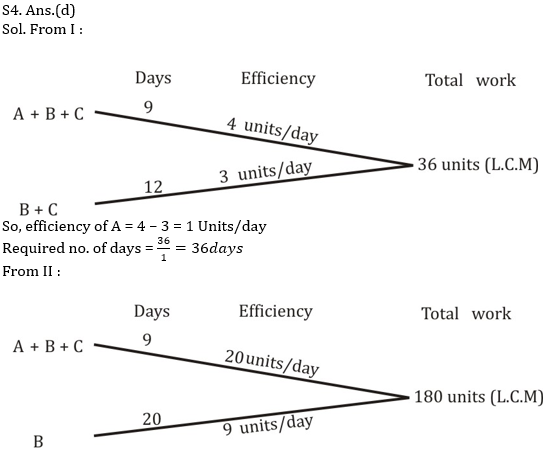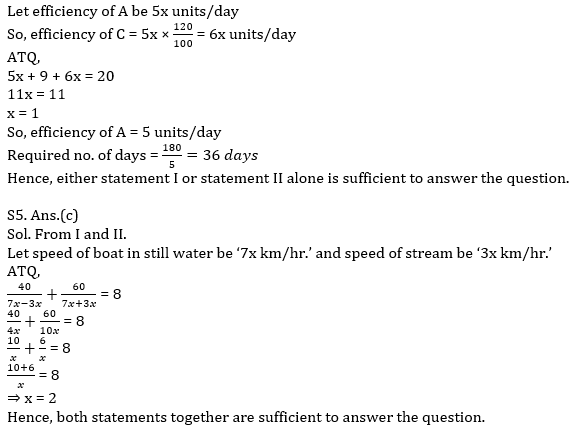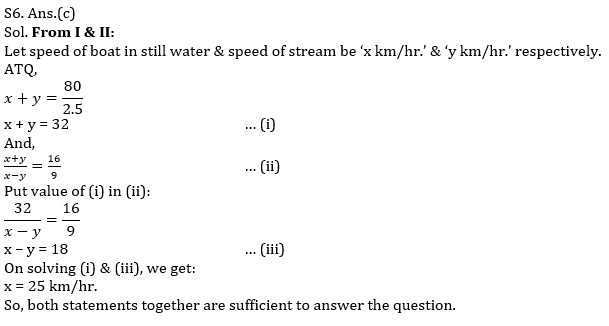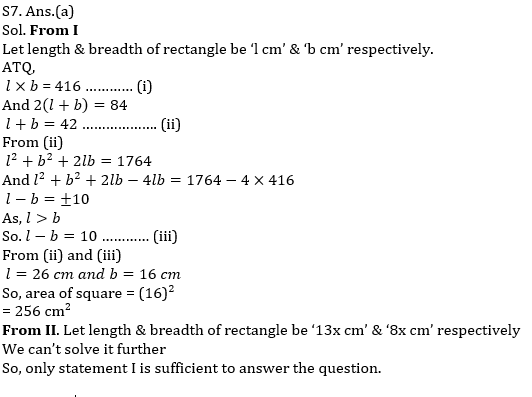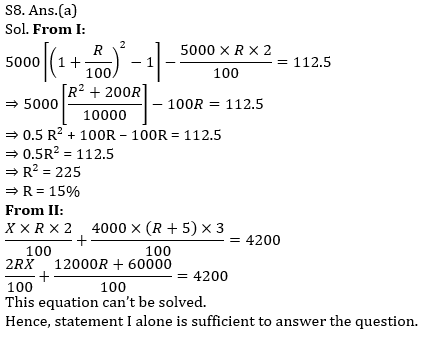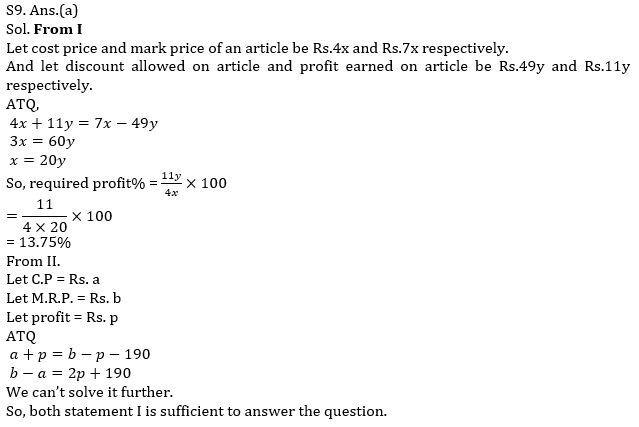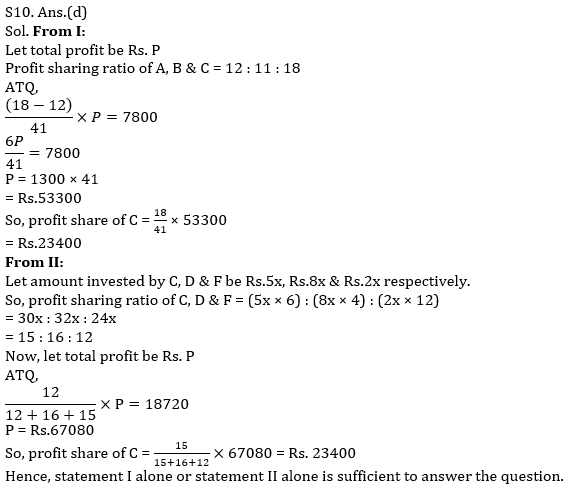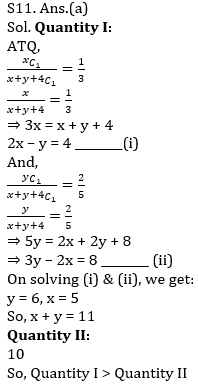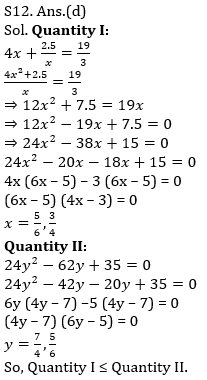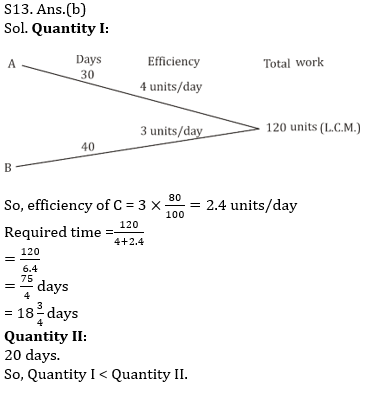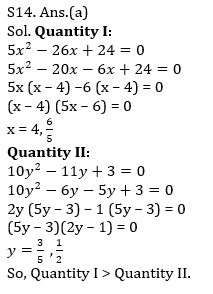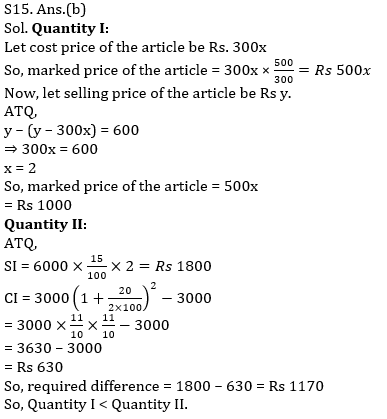×

Thank You, Your details have been submitted we will get back to you.Join India's largest learning destination

What You Will get ?

•Job Alerts
•Daily Quizzes
•Subject-Wise Quizzes
•Current Affairs
•Previous year question papers
•Doubt Solving session

ORJoin India's largest learning destination

What You Will get ?

•Job Alerts
•Daily Quizzes
•Subject-Wise Quizzes
•Current Affairs
•Previous year question papers
•Doubt Solving session

ORJoin India's largest learning destination

What You Will get ?

•Job Alerts
•Daily Quizzes
•Subject-Wise Quizzes
•Current Affairs
•Previous year question papers
•Doubt Solving session

Enter the email address associated with your account, and we'll email you an OTP to verify it's you.Join India's largest learning destination

What You Will get ?

•Job Alerts
•Daily Quizzes
•Subject-Wise Quizzes
•Current Affairs
•Previous year question papers
•Doubt Solving session

Enter OTP

Please enter the OTP sent to
/6

Did not recive OTP?

Resend in 60sJoin India's largest learning destination

What You Will get ?

•Job Alerts
•Daily Quizzes
•Subject-Wise Quizzes
•Current Affairs
•Previous year question papers
•Doubt Solving sessionJoin India's largest learning destination

What You Will get ?

•Job Alerts
•Daily Quizzes
•Subject-Wise Quizzes
•Current Affairs
•Previous year question papers
•Doubt Solving session

Almost there

+91Join India's largest learning destination

What You Will get ?

•Job Alerts
•Daily Quizzes
•Subject-Wise Quizzes
•Current Affairs
•Previous year question papers
•Doubt Solving session

Enter OTP

Please enter the OTP sent to Edit Number

Did not recive OTP?

Resend 60

By skipping this step you will not recieve any free content avalaible on adda247, also you will miss onto notification and job alerts

Are you sure you want to skip this step?

By skipping this step you will not recieve any free content avalaible on adda247, also you will miss onto notification and job alerts

Are you sure you want to skip this step?